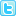ISSN: 0970-938X (Print) | 0976-1683 (Electronic)

# Biomedical Research

An International Journal of Medical Sciences

## Computational modeling of amperometric choline biosensor for neurochemical detection

The behavior of biosensors is based on the well-defined physical, chemical and biological reactions, which are expressed by nonlinear differential equations. The characteristics of diffusion reaction of choline biosensor are analyzed mathematically for wide range of input parameters such as initial concentration of choline and thickness of choline oxide enzyme layer. The mathematical simulations are done in MATLAB software using partial differential equation solver pdepe function. The analytical results are compared with numerically simulated results for various conditions to validate the model parameters. A numerical simulation result shows less than 2% deviation from analytical results for various substrate concentrations. For dimensionless diffusion distance x=0 and sigma=1, the dimensionless substrate and product concentrations are 0.72 and 0.18 when t=1 and changes exponentially with time.

Author(s): P Ravikumar, N Jeyashanthi, S Arumugam
Abstract | Full-Text | PDF

Share this